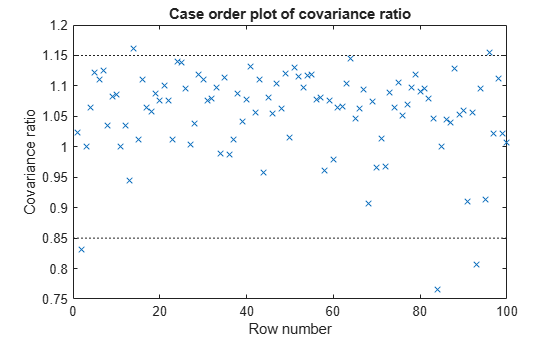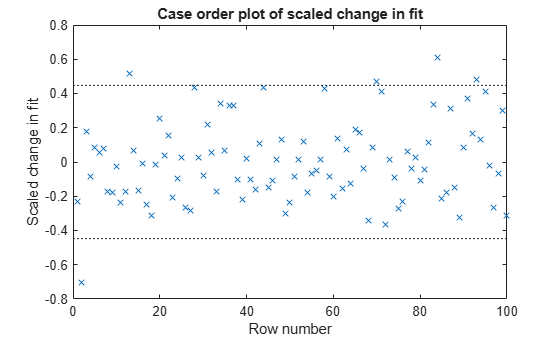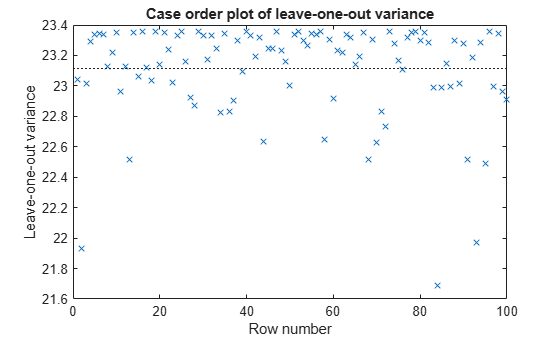## Delete-1 Statistics

### Delete-1 Change in Covariance (`CovRatio`)

#### Purpose

Delete-1 change in covariance (`CovRatio`) identifies the observations that are influential in the regression fit. An influential observation is one where its exclusion from the model might significantly alter the regression function. Values of `CovRatio` larger than 1 + 3*p/n or smaller than 1 – 3*p/n indicate influential points, where p is the number of regression coefficients, and n is the number of observations.

#### Definition

The `CovRatio` statistic is the ratio of the determinant of the coefficient covariance matrix with observation i deleted to the determinant of the covariance matrix for the full model:

`$\text{CovRatio}=\frac{\mathrm{det}\left\{MSE\left(i\right){\left[{X}^{\prime }\left(i\right)X\left(i\right)\right]}^{-1}\right\}}{\mathrm{det}\left[MSE{\left({X}^{\prime }X\right)}^{-1}\right]}.$`

`CovRatio` is an n-by-1 vector in the `Diagnostics` table of the fitted `LinearModel` object. Each element is the ratio of the generalized variance of the estimated coefficients when the corresponding element is deleted to the generalized variance of the coefficients using all the data.

#### How To

After obtaining a fitted model, say, `mdl`, using `fitlm` or `stepwiselm`, you can:

• Display the `CovRatio` by indexing into the property using dot notation

`mdl.Diagnostics.CovRatio`

• Plot the delete-1 change in covariance using

`plotDiagnostics(mdl,'CovRatio')`
For details, see the `plotDiagnostics` method of the `LinearModel` class.

#### Determine Influential Observations Using `CovRatio`

This example shows how to use the `CovRatio` statistics to determine the influential points in data. Load the sample data and define the response and predictor variables.

```load hospital y = hospital.BloodPressure(:,1); X = double(hospital(:,2:5));```

Fit a linear regression model.

`mdl = fitlm(X,y);`

Plot the `CovRatio` statistics.

`plotDiagnostics(mdl,'CovRatio')`For this example, the threshold limits are 1 + 3*5/100 = 1.15 and 1 - 3*5/100 = 0.85. There are a few points beyond the limits, which might be influential points.

Find the observations that are beyond the limits.

`find((mdl.Diagnostics.CovRatio)>1.15|(mdl.Diagnostics.CovRatio)<0.85)`
```ans = 5×1 2 14 84 93 96 ```

### Delete-1 Scaled Difference in Coefficient Estimates (`Dfbetas`)

#### Purpose

The sign of a delete-1 scaled difference in coefficient estimate (`Dfbetas`) for coefficient j and observation i indicates whether that observation causes an increase or decrease in the estimate of the regression coefficient. The absolute value of a `Dfbetas` indicates the magnitude of the difference relative to the estimated standard deviation of the regression coefficient. A `Dfbetas` value larger than 3/sqrt(n) in absolute value indicates that the observation has a large influence on the corresponding coefficient.

#### Definition

`Dfbetas` for coefficient j and observation i is the ratio of the difference in the estimate of coefficient j using all observations and the one obtained by removing observation i, and the standard error of the coefficient estimate obtained by removing observation i. The `Dfbetas` for coefficient j and observation i is

`$Dfbeta{s}_{ij}=\frac{{b}_{j}-{b}_{j\left(i\right)}}{\sqrt{MS{E}_{\left(i\right)}}\left(1-{h}_{ii}\right)},$`

where bj is the estimate for coefficient j, bj(i) is the estimate for coefficient j by removing observation i, MSE(i) is the mean squared error of the regression fit by removing observation i, and hii is the leverage value for observation i. `Dfbetas` is an n-by-p matrix in the `Diagnostics` table of the fitted `LinearModel` object. Each cell of `Dfbetas` corresponds to the `Dfbetas` value for the corresponding coefficient obtained by removing the corresponding observation.

#### How To

After obtaining a fitted model, say, `mdl`, using `fitlm` or `stepwiselm`, you can obtain the `Dfbetas` values as an n-by-p matrix by indexing into the property using dot notation,

`mdl.Diagnostics.Dfbetas`

#### Determine Observations Influential on Coefficients Using `Dfbetas`

This example shows how to determine the observations that have large influence on coefficients using `Dfbetas`. Load the sample data and define the response and independent variables.

```load hospital y = hospital.BloodPressure(:,1); X = double(hospital(:,2:5));```

Fit a linear regression model.

`mdl = fitlm(X,y);`

Find the `Dfbetas` values that are high in absolute value.

```[row,col] = find(abs(mdl.Diagnostics.Dfbetas)>3/sqrt(100)); disp([row col])```
``` 2 1 28 1 84 1 93 1 2 2 13 3 84 3 2 4 84 4 ```

### Delete-1 Scaled Change in Fitted Values (`Dffits`)

#### Purpose

The delete-1 scaled change in fitted values (`Dffits`) show the influence of each observation on the fitted response values. `Dffits` values with an absolute value larger than 2*sqrt(p/n) might be influential.

#### Definition

`Dffits` for observation i is

`${\text{Dffits}}_{i}=s{r}_{i}\sqrt{\frac{{h}_{ii}}{1-{h}_{ii}}},$`

where sri is the studentized residual, and hii is the leverage value of the fitted `LinearModel` object. `Dffits` is an n-by-1 column vector in the `Diagnostics` table of the fitted `LinearModel` object. Each element in `Dffits` is the change in the fitted value caused by deleting the corresponding observation and scaling by the standard error.

#### How To

After obtaining a fitted model, say, `mdl`, using `fitlm` or `stepwiselm`, you can:

• Display the `Dffits` values by indexing into the property using dot notation

`mdl.Diagnostics.Dffits`

• Plot the delete-1 scaled change in fitted values using

`plotDiagnostics(mdl,'Dffits')`
For details, see the `plotDiagnostics` method of the `LinearModel` class for details.

#### Determine Observations Influential on Fitted Response Using `Dffits`

This example shows how to determine the observations that are influential on the fitted response values using `Dffits` values. Load the sample data and define the response and independent variables.

```load hospital y = hospital.BloodPressure(:,1); X = double(hospital(:,2:5));```

Fit a linear regression model.

`mdl = fitlm(X,y);`

Plot the `Dffits` values.

`plotDiagnostics(mdl,'Dffits')`The influential threshold limit for the absolute value of `Dffits` in this example is 2*sqrt(5/100) = 0.45. Again, there are some observations with `Dffits` values beyond the recommended limits.

Find the `Dffits` values that are large in absolute value.

`find(abs(mdl.Diagnostics.Dffits)>2*sqrt(4/100))`
```ans = 10×1 2 13 28 44 58 70 71 84 93 95 ```

### Delete-1 Variance (`S2_i`)

#### Purpose

The delete-1 variance (`S2_i`) shows how the mean squared error changes when an observation is removed from the data set. You can compare the `S2_i` values with the value of the mean squared error.

#### Definition

`S2_i` is a set of residual variance estimates obtained by deleting each observation in turn. The `S2_i` value for observation i is

`$S2_i=MS{E}_{\left(i\right)}=\frac{\sum _{j\ne i}^{n}{\left[{y}_{j}-{\stackrel{^}{y}}_{j\left(i\right)}\right]}^{2}}{n-p-1},$`

where yj is the jth observed response value. `S2_i` is an n-by-1 vector in the `Diagnostics` table of the fitted `LinearModel` object. Each element in `S2_i` is the mean squared error of the regression obtained by deleting that observation.

#### How To

After obtaining a fitted model, say, `mdl`, using `fitlm` or `stepwiselm`, you can:

• Display the `S2_i` vector by indexing into the property using dot notation

`mdl.Diagnostics.S2_i`

• Plot the delete-1 variance values using

`plotDiagnostics(mdl,'S2_i')`
For details, see the `plotDiagnostics` method of the `LinearModel` class.

#### Compute and Examine Delete-1 Variance Values

This example shows how to compute and plot S2_i values to examine the change in the mean squared error when an observation is removed from the data. Load the sample data and define the response and independent variables.

```load hospital y = hospital.BloodPressure(:,1); X = double(hospital(:,2:5));```

Fit a linear regression model.

`mdl = fitlm(X,y);`

Display the MSE value for the model.

`mdl.MSE`
```ans = 23.1140 ```

Plot the S2_i values.

`plotDiagnostics(mdl,'S2_i')`This plot makes it easy to compare the S2_i values to the MSE value of 23.114, indicated by the horizontal dashed lines. You can see how deleting one observation changes the error variance.